Practice the questions of McGraw Hill Math Grade 4 Answer Key PDF Chapter 6 Test to secure good marks & knowledge in the exams.

Find each quotient and remainder. You can draw pictures for each problem if you need help.

Question 1.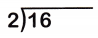Explanation:
quotient is 8
remainder is 8

Question 2.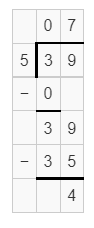Explanation:
quotient is 7
remainder is 4

Question 3.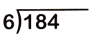Explanation:
quotient is 30
remainder is 4

Question 4.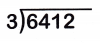Explanation:
quotient is 2137
remainder is 1

Question 5.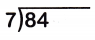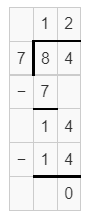Explanation:
quotient is 12
remainder is 0

Question 6.Explanation:
quotient is 22
remainder is 3

Question 7.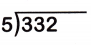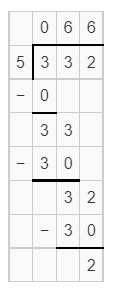Explanation:
quotient is 66
remainder is 2

Question 8.Explanation:
quotient is 993
remainder is 3

Question 9.Explanation:
quotient is 9
remainder is 0

Question 10.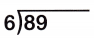Explanation:
quotient is 14
remainder is 5

Question 11.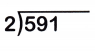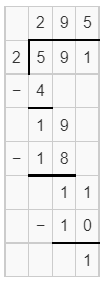Explanation:
quotient is 295
remainder is 1

Question 12.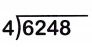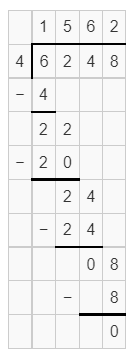Explanation:
quotient is 1562
remainder is 0

Use mental math to find each quotient.

Question 13.
600 ÷ 6 = ___
600 ÷ 6 = 100
Explanation:
6 ÷ 6 = 1
6 x 1 = 6
so, we have to add the remaining zeros

Question 14.
540 ÷ 9 = ___
540 ÷ 9 = 60
Explanation:
54 ÷ 9 = 6
9 x 6 = 54
9 x 6 = 54
so, we have to add the remaining zeros

Question 15.
320 ÷ 4 = ___
320 ÷ 4 = 80
Explanation:
32 ÷ 4 = 8
8 x 4 = 32
so, we have to add the remaining zeros

Estimate each quotient.

Question 16.
181 ÷ 3 = ___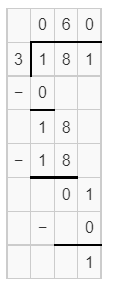Explanation:
quotient is 60
remainder is 1

Question 17.
452 ÷ 5 = ___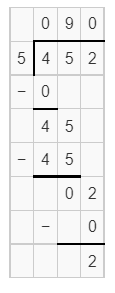Explanation:
quotient is 90
remainder is 2

Question 18.
876 ÷ 8 = ___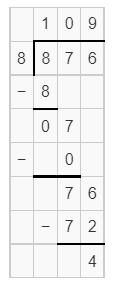Explanation:
quotient is 109
remainder is 4

Solve. If you divide, explain what the remainder shows.

Question 19.
A deli has 5 trays of sandwiches. There are 6 sandwiches on each tray. How many sandwiches does the deli have?
___________________
The manager places the sandwiches on 4 shelves. He puts an equal number of sandwiches on each shelf. How many sandwiches does he put on each shelf?
he kept 5 sandwiches in shelves.
Explanation:
5 trays of sandwiches.
6 sandwiches on each tray.
5 x 6 = 30
30 ÷  6 = 5

Question 20.
Scott has 348 comic books. He places the comic books into 8 boxes. He puts an equal number of comic books in each box. How many comic books are in each box?Explanation:
Scott has 348 comic books.
He places the comic books into 8 boxes.
43 comic books in each box.

Question 21.
639 people see a movie on Saturday. 711 people see the movie on Sunday. How many people saw the movie in all?
_________________________
427 people bought popcorn during the movie. How many people did not buy popcorn during the movie?
1350 people saw the movie
932 people didn’t buy the popcorn
Explanation:
639 on Saturday
711 on Sunday
639 + 711 = 1350